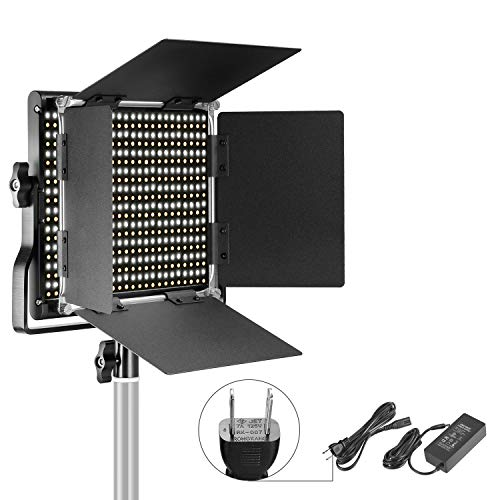## NEEWER LED-NL660 というのを買ってみたNeewer 調光可能な二色660 LEDビデオライト 耐久性のあるメタルフレーム、 Uブラケットと遮光板付き 3200-5600K、CRI96+ スタジオ撮影、YouTube、商品撮影、ビデオ撮影に適用 NEEWER ★ 4.0 / 5.0 cho45

NEEWER LED-NL660 というものをタイムセールで買ってみたので ColorMunki Photo + Argyll CMS で測定してみた。

WHITE と YELLOW というつまみがあり、2種類のLEDの光量が変更できるようになっている。色温度の調整はこれを微妙に動かして行う。

### WHITE 全部

 Result is XYZ: 5747.510694 6065.655076 5528.248292, D50 Lab: 439.775063 -11.375823 -26.558254
Ambient = 6065.7 Lux, CCT = 5545K (Duv 0.0049)
Suggested EV @ ISO100 for 6065.7 Lux incident light = 11.2
Closest Planckian temperature = 5376K (DE2K 6.5)
Closest Daylight temperature  = 5492K (DE2K 2.3)
Color Rendering Index (Ra) = 97.5 [ R9 = 85.5 ]
R1  = 97.0  R2  = 98.3  R3  = 99.7  R4  = 98.1  R5  = 97.6  R6  = 96.7  R7  = 97.9
R8  = 94.6  R9  = 85.5  R10 = 97.6  R11 = 97.7  R12 = 83.7  R13 = 97.5  R14 = 98.4
Television Lighting Consistency Index 2012 (Qa) = 98.6

スペック上は5600Kまでと書いてあるが 5500K の LED っぽい。

### YELLOW 全部

 Result is XYZ: 5563.515641 5243.322549 2120.666499, D50 Lab: 418.170369 60.679109 158.296845
Ambient = 5243.3 Lux, CCT = 3126K (Duv 0.0016)
Suggested EV @ ISO100 for 5243.3 Lux incident light = 11.0
Closest Planckian temperature = 3079K (DE2K 3.0)
Closest Daylight temperature  = 3095K (DE2K 0.9)
Color Rendering Index (Ra) = 98.1 [ R9 = 88.9 ]
R1  = 99.0  R2  = 99.3  R3  = 99.0  R4  = 98.8  R5  = 98.5  R6  = 98.1  R7  = 97.3
R8  = 95.0  R9  = 88.9  R10 = 98.3  R11 = 98.5  R12 = 85.5  R13 = 99.2  R14 = 98.6
Television Lighting Consistency Index 2012 (Qa) = 98.1

3100Kぐらいが下限

### WHITE+YELLOW 全部

 Result is XYZ: 11395.644074 11395.813163 7722.945028, D50 Lab: 546.392814 29.623320 61.495553
Ambient = 11395.8 Lux, CCT = 4173K (Duv 0.0006)
Suggested EV @ ISO100 for 11395.8 Lux incident light = 12.2
Closest Planckian temperature = 4158K (DE2K 0.9)
Closest Daylight temperature  = 4232K (DE2K -3.6)
Color Rendering Index (Ra) = 98.8 [ R9 = 96.1 ]
R1  = 99.3  R2  = 99.6  R3  = 99.6  R4  = 99.6  R5  = 98.9  R6  = 97.3  R7  = 98.4
R8  = 97.8  R9  = 96.1  R10 = 99.1  R11 = 97.1  R12 = 83.4  R13 = 99.2  R14 = 98.5
Television Lighting Consistency Index 2012 (Qa) = 98.3

### 5000K

5000K 程度にあわせたとき。(WHITE 全部+YELLOWが左水平とちょっとぐらい)

 Result is XYZ: 6934.349461 7181.415867 5994.476376, D50 Lab: 466.163889 1.000754 -3.284202
Ambient = 7181.4 Lux, CCT = 5029K (Duv 0.0029)
Suggested EV @ ISO100 for 7181.4 Lux incident light = 11.5
Closest Planckian temperature = 4941K (DE2K 4.1)
Closest Daylight temperature  = 5042K (DE2K -0.6)
Color Rendering Index (Ra) = 98.2 [ R9 = 90.1 ]
R1  = 98.4  R2  = 99.3  R3  = 99.5  R4  = 98.5  R5  = 99.0  R6  = 97.0  R7  = 97.9
R8  = 96.0  R9  = 90.1  R10 = 99.3  R11 = 96.9  R12 = 85.9  R13 = 99.0  R14 = 98.7
Television Lighting Consistency Index 2012 (Qa) = 98.6

Ra は全ての指定色でかなり高い値が出てる。R12(青)もそこそこ高い値が出ていて良い。

このときのスペクトル

## Node.js の fs モジュールだけで GPIO の割込みを扱うには？

select や poll を明示的に呼び出すことができないが、edge を設定しさえすれば fs.watch でイベントを受けとれるようだ。

また、seek() もなぜか存在してないが read() の引数に position というのがあり、これが seek() 相当になるようだ。

const fs_ = require('fs');
const fs = fs_.promises;
fs.watch = fs_.watch;

async function watchInterrupt(pin, func) {
try {
await fs.writeFile(/sys/class/gpio/export, ${pin}); } catch (e) { if (e.code === 'EBUSY') { // ignore } else { throw e; } } await fs.writeFile(/sys/class/gpio/gpio${pin}/direction, 'in');
await fs.writeFile(/sys/class/gpio/gpio${pin}/edge, 'both'); // falling raising both const fh = await fs.open(/sys/class/gpio/gpio${pin}/value, 'r');
const buf = new Uint8Array(1);
fh.read(buf, 0, 1, 0);
const watcher = fs.watch(/sys/class/gpio/gpio${pin}/value, {}, (eventType, filename) => { if (eventType === "change") { fh.read(buf, 0, 1, 0); const val = buf-48; func(val, pin); } else { // XXX console.log(unchecked event "${eventType}" occured with "${filename}"); } }); return { close: async () => { watcher.close(); fh.close(); await fs.writeFile(/sys/class/gpio/gpio${pin}/edge, 'none');
}
};
}

(async () => {
watchInterrupt(0, (val, pin) => {
console.log(gpio${pin} changed ->${val});
});
watchInterrupt(2, (val, pin) => {
console.log(gpio${pin} changed ->${val});
});
watchInterrupt(3, (val, pin) => {
console.log(gpio${pin} changed ->${val});
});
})();